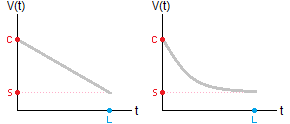# How to Calculate Asset Depreciation

## Exponential and Straight Line Depreciation

Asset Depreciation Calculator
linear                      exponentialPurchase Price \$
Scrap Value \$
Lifespan      years

Depreciation models are mathematical formulas that tell you how much your assets will be worth in a certain number of years. Businesses use asset depreciation to set budgets, plan for future expenses, and appraise the total value of their holdings.

There are several different techniques to determine the loss of value; we will discuss two. (1) Straight line depreciation, which assumes that the asset decreases by a fixed dollar amount every year until the asset reaches its salvage value. (2) Exponential depreciation (a type of curved, non-linear, or accelerated depreciation) which is based on a fixed annual percent decrease.

Each model will give you slightly different values, but both are valuable tools that business owners should understand. You can compute the depreciation manually with the formula below, or use the calculator on the left.

### Straight Line Depreciation

To determine the straight line decline in an asset's value, you must know its initial cost (purchase price) C, its average lifespan L, and its salvage value S. The salvage value may be zero, but most things can be sold for scrap, or repurposed to some extent.

If V(t) is the function that gives the assets value after t years, then

V(t) = C - [(C-S)/L]t.

Example: Suppose your company car was purchased for \$12,000, its estimated useful lifespan is 10 years, and its salvage value is \$2,500. Then the straight line depreciation model is

V(t) = 12000 - 950t.

Essentially, this model predicts that the car's value will decrease by \$950 every year. After 5 years, it will be worth 12000 - (950)(5) = \$7,250. After 10 or more years it will be worth 12000 - (950)(10) = \$2,500.

### Curved or Exponential Depreciation (Annual % Decrease)

To determine the annual percent decrease of the asset's value, you need to know the purchase price C, approximate lifespan L, and salvage value S. The annual percent change is given by the formula

100% - [(S/C)(1/L)](100%).

The value function V(t) is

V(t) = C[(S/C)(1/L)]t.

Example: Let us use the same example of the company car as above, but this time assume that the depreciation is accelerated. The annual percent decrease in its value is

100% - [(2500/12000)(1/10)](100%)
= 100% - [(0.20833)0.1](100%)
= 100% - [0.855](100%)
= 100% - 85.5%
= 14.5%, so it loses 14.5% of its value every year.

The depreciation function is

V(t) = 12000[0.855]t.

The value of the car in 5 years will be 12000(0.855)5 = \$5,483 with this model.

Note: Nowadays, there are more accurate methods for calculating the depreciation of a car, one of them is presented here.